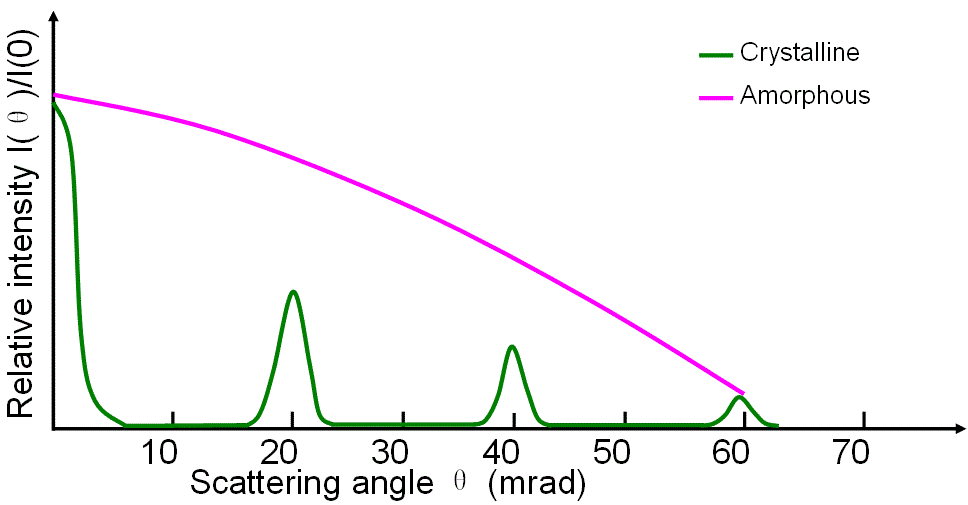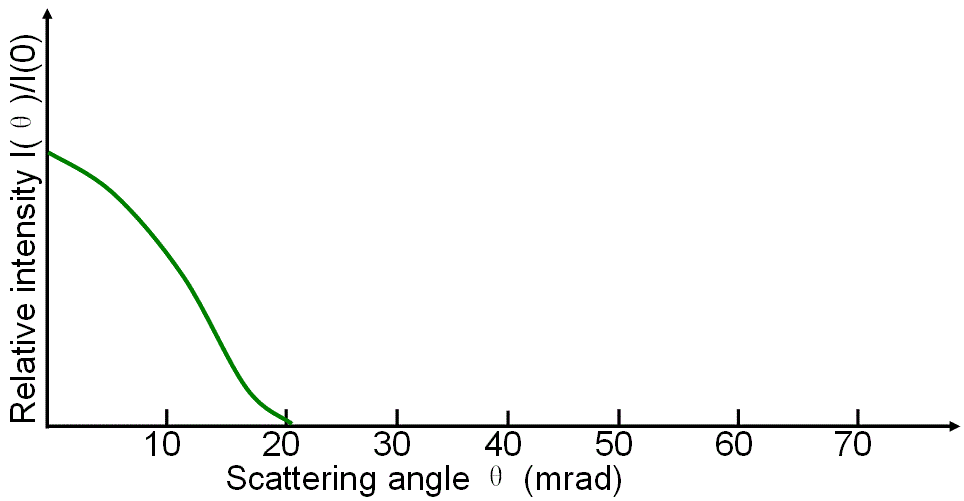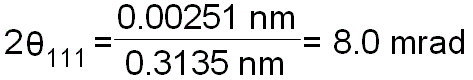Elastic Scattering Angle (& diffraction angle) of Electrons
- Practical Electron Microscopy and Database -
- An Online Book -Microanalysis | EM Book                                                                   https://www.globalsino.com/EM/

Rutherford cross-section gives convenient formulas to calculate the elastic scattering angle and total elastic cross section so that it is often used to describe the elastic scattering of electron. However, its accuracy becomes worse in low-energy region or in high atomic-number materials. As atomic number (z) increases the elastic scattering cross section becomes larger and thus the possibility of scattering into large angles becomes higher which increases the backscattering coefficient.

In general, the inelastic scattering of electrons occurs at smaller angles than that for elastic scattering. Figure 4754 shows angular distributions of various scattered electrons as a function of scattering angle in EMs.(a) (b)(c) (d)

Figure 4754. (a) Elastic scattering of electrons with crystalline and amorphous specimens, (b) Phonon scattering, (c) Inner-shell ionization losses, and (d) Plasmon losses.

For crystals, for instance, the wavelength of 200-kV TEM is 0.00251 nm, and d111 for Si is 0.3135 nm, thus the angle between direct beam and Si 111 spot is given by (see page3580),------------------- 

 ;